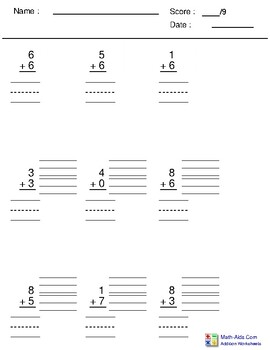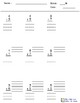# Single Digit Addition (vertical) #1Subject
Resource Type
File Type

PDF

(447 KB|10 pages)
Product Rating
Standards
• Product Description
• StandardsNEW

This is 10 pages of vertical addition problems using the digits 0-9. They are from www.math-aids.com, but I have modified them so that there are 9 problems on each page (3 across and 3 down), there is a ten-frame next to each digit in the math problem, and there are lines for the student to write their answer on. I have also modified the top of the worksheet so that there are the following lines:

Name: ______ Score: ___/9

Date: ______

THIS IS THE FIRST OF 3 SETS OF 10 PAGES EACH

Add and subtract within 20, demonstrating fluency for addition and subtraction within 10. Use strategies such as counting on; making ten (e.g., 8 + 6 = 8 + 2 + 4 = 10 + 4 = 14); decomposing a number leading to a ten (e.g., 13 - 4 = 13 - 3 - 1 = 10 - 1 = 9); using the relationship between addition and subtraction (e.g., knowing that 8 + 4 = 12, one knows 12 - 8 = 4); and creating equivalent but easier or known sums (e.g., adding 6 + 7 by creating the known equivalent 6 + 6 + 1 = 12 + 1 = 13).
Relate counting to addition and subtraction (e.g., by counting on 2 to add 2).
Fluently add and subtract within 5.
Represent addition and subtraction with objects, fingers, mental images, drawings, sounds (e.g., claps), acting out situations, verbal explanations, expressions, or equations.
Compose and decompose numbers from 11 to 19 into ten ones and some further ones, e.g., by using objects or drawings, and record each composition or decomposition by a drawing or equation (e.g., 18 = 10 + 8); understand that these numbers are composed of ten ones and one, two, three, four, five, six, seven, eight, or nine ones.
Total Pages
10 pages
N/A
Teaching Duration
N/A
Report this Resource to TpT
Reported resources will be reviewed by our team. Report this resource to let us know if this resource violates TpT’s content guidelines.